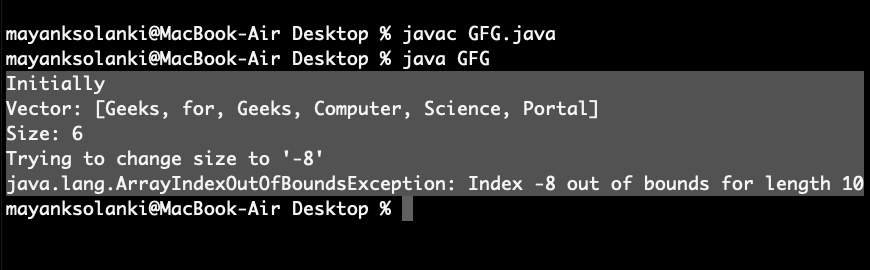# Vector setSize() method in Java with Example

The Java.util.Vector.setSize() is a method of Vector class that is used to set the new size of the vector. If the new size of the vector is greater than the current size then null elements are added to the vector is new size is less than the current size then all higher-order elements are deleted.

This method modified the size of the Vector to the newSize and does not return anything.

Syntax:

`public void setSize(int newSize)`

Parameters: This method accepts a mandatory parameter newSize of the vector.

Return Type: NA

Exceptions: ArrayIndexOutOfBoundsException

Note: If new size is negative then it will throw Runtime Error

Example 1:

## Java

 `// Java Program to Illustrate setSize() method` `// of Vector class`   `// Importing required classes` `import` `java.util.*;`   `// Main class` `public` `class` `GFG {`   `    ``// Main driver method` `    ``public` `static` `void` `main(String[] args)` `    ``{`   `        ``// Creating object of Vector class` `        ``// Declaring object of string type` `        ``Vector v = ``new` `Vector();`   `        ``// Inserting elements into the vector` `        ``// using add() method` `        ``// Custom input elements` `        ``v.add(``"Geeks"``);` `        ``v.add(``"for"``);` `        ``v.add(``"Geeks"``);` `        ``v.add(``"Computer"``);` `        ``v.add(``"Science"``);` `        ``v.add(``"Portal"``);`   `        ``// Printing vector before calling setSize() method` `        ``System.out.println(``"Initially"``);` `        ``System.out.println(``"Vector: "` `+ v);` `        ``System.out.println(``"Size: "` `+ v.size());`   `        ``// Setting new custom size` `        ``v.setSize(``8``);`   `        ``// Printing vector after calling setSize()` `        ``System.out.println(``"\nAfter using setSize()"``);` `        ``System.out.println(``"Vector: "` `+ v);` `        ``System.out.println(``"Size: "` `+ v.size());` `    ``}` `}`

Output

```Initially
Vector: [Geeks, for, Geeks, Computer, Science, Portal]
Size: 6

After using setSize()
Vector: [Geeks, for, Geeks, Computer, Science, Portal, null, null]
Size: 8```

Example 2: When new size is positive

## Java

 `// Java program to Illustrate setSize() method` `// of Vector class` `// Where size is positive `   `// Importing utility classes` `import` `java.util.*;`   `// Main class` `public` `class` `GFG {`   `    ``// amin driver method` `    ``public` `static` `void` `main(String[] args)` `    ``{` `        ``// Creating vector object of string type` `        ``Vector v = ``new` `Vector();`   `        ``// Inserting elements into the vector` `        ``//. using add() method` `        ``// Custom input elements` `        ``v.add(``"Geeks"``);` `        ``v.add(``"for"``);` `        ``v.add(``"Geeks"``);` `        ``v.add(``"Computer"``);` `        ``v.add(``"Science"``);` `        ``v.add(``"Portal"``);`   `        ``// Printing vector before calling setSize()` `        ``System.out.println(``"Initially"``);` `        ``System.out.println(``"Vector: "` `+ v);` `        ``System.out.println(``"Size: "` `+ v.size());`   `        ``// Setting new size` `        ``v.setSize(``4``);`   `        ``// Printing vector after calling setSize()` `        ``System.out.println(``"\nAfter using setSize()"``);` `        ``System.out.println(``"Vector: "` `+ v);` `        ``System.out.println(``"Size: "` `+ v.size());` `    ``}` `}`

Output

```Initially
Vector: [Geeks, for, Geeks, Computer, Science, Portal]
Size: 6

After using setSize()
Vector: [Geeks, for, Geeks, Computer]
Size: 4```

Example 3: When the new size is negative

## Java

 `// Java program to Illustrate setSize() method` `// of vector class` `// Where size is negative`   `// Importing utility classes` `import` `java.util.*;`   `// Main class` `public` `class` `GFG {`   `    ``// Main driver method` `    ``public` `static` `void` `main(String[] args)` `    ``{` `        ``// Creating Vector class object of string type` `        ``Vector v = ``new` `Vector();`   `        ``// Inserting elements into the vector` `        ``// using add() method` `        ``v.add(``"Geeks"``);` `        ``v.add(``"for"``);` `        ``v.add(``"Geeks"``);` `        ``v.add(``"Computer"``);` `        ``v.add(``"Science"``);` `        ``v.add(``"Portal"``);`   `        ``// Printing vector before calling setSize()` `        ``System.out.println(``"Initially"``);` `        ``System.out.println(``"Vector: "` `+ v);` `        ``System.out.println(``"Size: "` `+ v.size());`   `        ``// Try block to check for exceptions` `        ``try` `{`   `            ``// Setting new size` `            ``v.setSize(-``8``);` `        ``}`   `        ``// Catch block to handle exceptions` `        ``catch` `(Exception e) {`   `            ``// Display message when exceptions occurred` `            ``System.out.println(``"Trying to change "` `                               ``+ ``"size to '-8'\n"` `+ e);` `        ``}` `    ``}` `}`

Output:Whether you're preparing for your first job interview or aiming to upskill in this ever-evolving tech landscape, GeeksforGeeks Courses are your key to success. We provide top-quality content at affordable prices, all geared towards accelerating your growth in a time-bound manner. Join the millions we've already empowered, and we're here to do the same for you. Don't miss out - check it out now!

Previous
Next